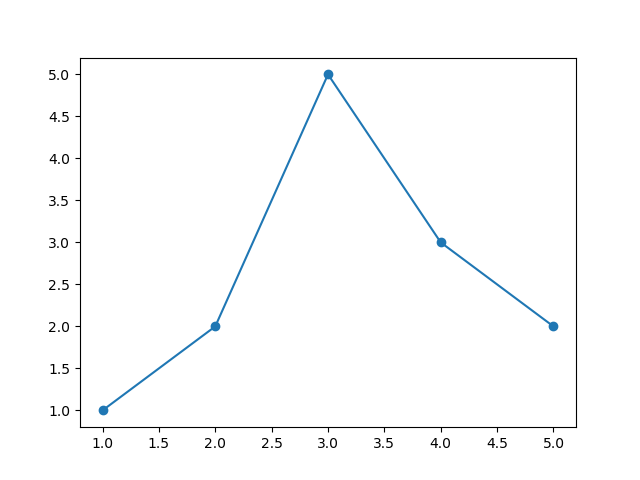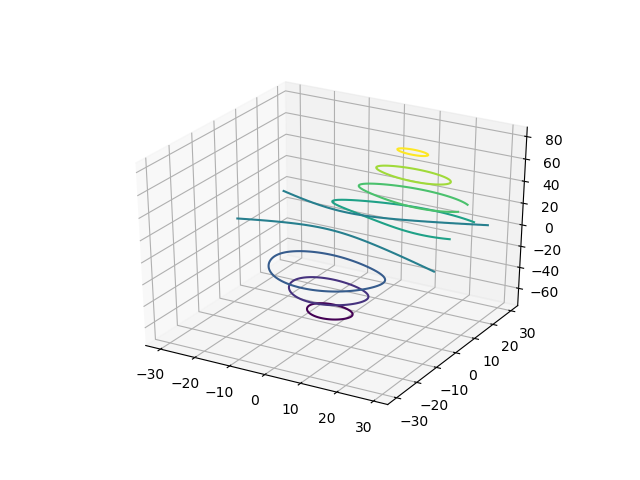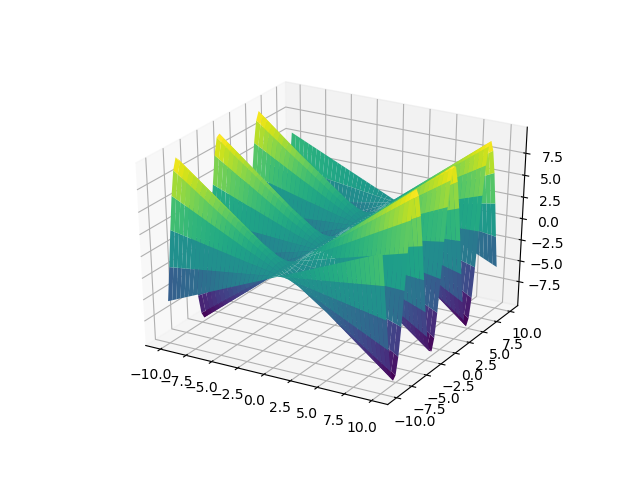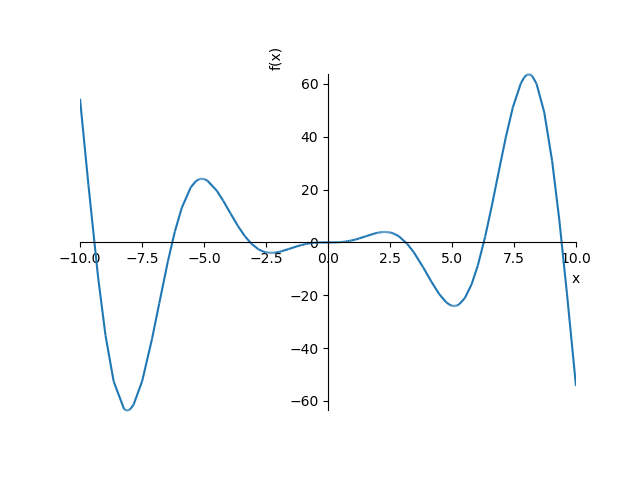a field-theory motivated approach to computer algebra

# Plotting data and functions

This notebook shows how to make plots of data and functions. Cadabra notebooks can display plots which are generated by matplotlib, so you have the full machinery of that library at your disposal to visualise your maths.

## Basic plotting with matplotlib

The first example that we will show is a basic matplotlib plot of a number of data points. You can find examples of this on any matplotlib tutorial. The only difference is how you make the plot appear in your Cadabra notebook. We start by generating the plot and storing it in a variable $p$.
import matplotlib.pyplot as plt import numpy as np p=plt.plot([1,2,3,4,5],[1,2,5,3,2],'-o')
We then make it appear in the notebook using the display command. This command figures out the type of the object which you hand it, and sends the appropriate data to the notebook interface.
display(p)## 3D plotting with matplotlib

from mpl_toolkits.mplot3d import axes3d import matplotlib.pyplot as plt fig=plt.figure() ax=fig.add_subplot(111,projection='3d') X,Y,Z = axes3d.get_test_data(0.05) cset = ax.contour(X,Y,Z) ax.clabel(cset,fontsize=9,inline=1)
display(fig)## Plotting functions with sympy

In order to plot symbolic functions, rather than numerical data, use sympy. This library can generate both two-dimensional and three-dimensional plots.
from sympy.plotting import plot, plot3d x,y=symbols('x y') p1=plot3d(sin(x)*y) p2=plot(sin(x)*x**2)
display(p1) display(p2)print(p1)
cartesian surface: y*sin(x) for y over (-10.0, 10.0) and x over (-10.0, 10.0)

print(repr(p1._backend.fig))
<matplotlib.figure.Figure object at 0x7f4e59637e80>